nextnano.com  GUI: nextnanomat  Tool: nextnano++  Tool: nextnano³  Tool: nextnano.NEGF  Download | Search | Copyright | News | Publications  * password protected nextnano³ software3D exciton/biexciton in cubic QD

# nextnano3 - Tutorial

## Exciton and biexciton corrections in idealistic 3D cubic quantum dots

Author: Stefan Birner

If you want to obtain the input files that are used within this tutorial, please contact stefan.birner@nextnano.de.
```-> 3DcubicQD_exciton_04nm.in   - ```4 nm x 4 nm x 4 nm QD``` -> 3DcubicQD_exciton_06nm.in   - ```6 nm x 6 nm x 6 nm QD``` -> 3DcubicQD_exciton_08nm.in   - ```8 nm x 8 nm x 8 nm QD``` -> 3DcubicQD_exciton_10nm.in   - ```10 nm x 10 nm x 10 nm QD``` -> 3DcubicQD_exciton_12nm.in   - ```12 nm x 12 nm x 12 nm QD``` -> 3DcubicQD_exciton_16nm.in   - ```16 nm x 16 nm x 16 nm QD``` -> 3DcubicQD_exciton_20nm.in   - ```20 nm x 20 nm x 20 nm QD``` -> 3DcubicQD_biexciton_04nm.in - ```4 nm x 4 nm x 4 nm QD``` -> 3DcubicQD_biexciton_06nm.in - ```6 nm x 6 nm x 6 nm QD``` -> 3DcubicQD_biexciton_08nm.in - ```8 nm x 8 nm x 8 nm QD``` -> 3DcubicQD_biexciton_10nm.in - ```10 nm x 10 nm x 10 nm QD``` -> 3DcubicQD_biexciton_12nm.in - ```12 nm x 12 nm x 12 nm QD``` -> 3DcubicQD_biexciton_16nm.in - ```16 nm x 16 nm x 16 nm QD``` -> 3DcubicQD_biexciton_20nm.in - ```20 nm x 20 nm x 20 nm QD```  ```

### Exciton correction in idealistic 3D cubic quantum dots

• Here, we want to calculate the correction of the electron-hole transition energies due to the exciton correction (Coulomb interaction of electron and hole).

• The binding of the electron and the hole in a quantum dot is primarily due to the conduction and valence band confinement. Thus the Coulomb interaction between these two particles is just a small correction to the one-particle energies. The magnitude of this energetic correction strongly depends on the size of the quantum dot and the height of the confining barriers. Thus one would expect a 1/r dependence (Coulomb potential) of the exciton correction in the quantum dot.

• To test this, we calculate the exciton correction in an idealistic cubic 3D InAs quantum dot. The band gap is assumed to be Egap = 0.417eV (corresponding to a temperature of 0 K). The grid resolution was taken to be 0.5 nm, apart from the 20 nm dot where we took a grid resolution of 1 nm in order to reduce CPU time.
We assumed infinite potential barriers at the quantum dot boundaries which correspond to Dirichlet boundary conditions for the wave functions at the quantum dot boundaries.

• To keep things simple, we assume isotropic and parabolic effective masses for the electron and heavy hole:
``` conduction-band-masses = 0.026d0 0.026d0 0.026d0 ! ```electron mass at Gamma conduction band```                          ... valence-band-masses    = 0.41d0  0.41d0  0.41d0  ! ```heavy hole mass```                          ...  ```
• The static dielectric constant is defined as follows:
``` static-dielectric-constants = 15.15d0 15.15d0 15.15d0 ! ```epsilon(0)

• Following the notation of the PhD thesis of O. Stier (TU Berlin) we have to solve:

( H + Ve ) Psih = E'h Psih
( H + Vh ) Psie = E'e Psie

The exciton has the following ground state energy: EX = E'e `-` E'h + C

C   =  ( < Psih | Ve | Psih > `-` < Psie | Vh | Psie > ) / 2

The potentials Ve and Vh are obtained from the relevant Poisson equations.

`-` exciton recombination energy: EX
(order of magnitude: ~ eV)
`-` exciton binding energy: The difference in energy between the noninteracting single-particle states and the exciton energy: Ee `-` Eh `-` EX
(order of magnitude: ~ meV)
• The following figures show an isosurface and contour plots of 2D slices through the ground state electron wave function (psi²) of the 20 nm cubic InAs quantum dot (without excitonic effects).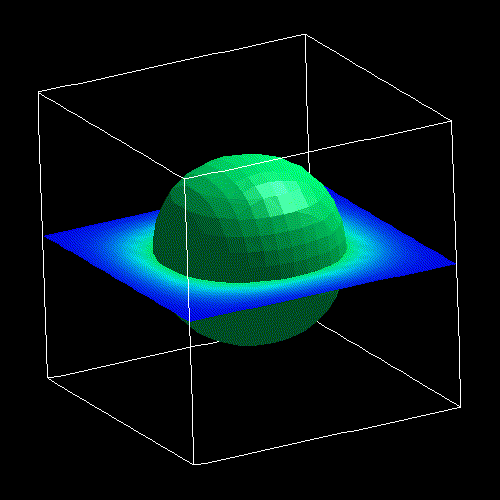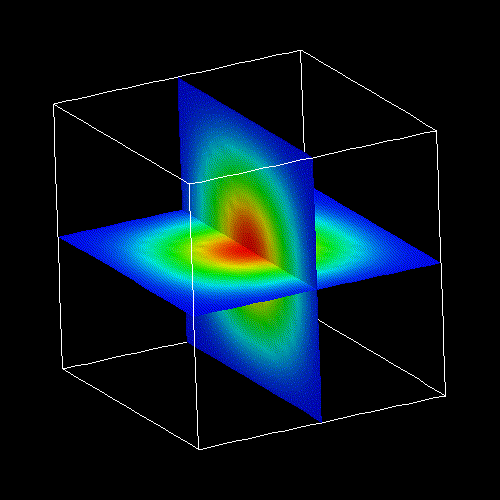• The following flags are needed to include the exciton corrections:

``` \$numeric-control  ...  !---------  ! Exciton  !---------  coulomb-matrix-element                = yes  ! 'yes' or 'no'  calculate-exciton                     = yes  ! 'yes' or 'no'  exciton-iterations                    = 10   ! e.g. 5    ```(for self-consistency loop)```  exciton-residual                      = 1d-6 ! e.g. 1d-5 ```(for self-consistency loop)```  exciton-electron-state-number         = 1    ! 1 = ```electron ground state```  exciton-hole-state-number             = 1    ! 1 = ```hole ground state```  !--------------------  ! Exciton: X0  !--------------------  number-of-electron-states-for-exciton = 1    ! ```electron ground state```  number-of-hole-states-for-exciton     = 1    ! ```hole ground state

• The energy of the exciton correction can be found in the file``` Schroedinger_1band/exciton_energy3Dcb001_vb001.dat ```in the column``` Delta_Ex ```in units of [eV].

• The following figure shows the exciton correction due to the Coulomb iteraction of the lowest electron state with the highest heavy hole state in cubic InAs quantum dots as a function of quantum dot size (4 nm / 6 nm / 8 nm / 10 nm / 12 nm / 16 nm / 20 nm).
The curve clearly shows a 1/r dependence as expected from the Coulomb term (indicated by the fitted curve).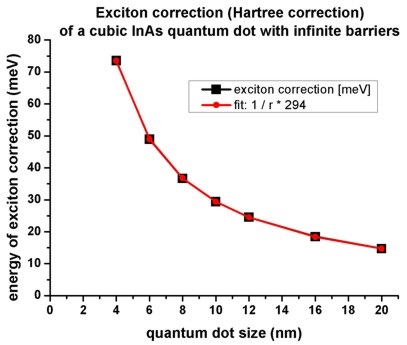• In addition to the correction in the electron and hole energies, the wave functions will also change. This affects the transition matrix elements. Due to the mutual attraction of electron and hole, the overlap of the wave functions increases. This also reduces the influence of externally applied electric fields (e.g. Quantum confined Stark shift).

### Biexciton correction in idealistic 3D cubic quantum dots

• The biexciton consists of two electrons and two holes.

```  exciton-electron-state-number         = 1   ! ```electron ground state```   exciton-hole-state-number             = 1   ! ```hole      ground state```   !--------------------  ! Biexciton: XX0  !--------------------  number-of-electron-states-for-exciton = 2   ! ```(This is still the electron ground state due to two-fold spin degeneracy.)```  number-of-hole-states-for-exciton     = 2   ! ```(This is still the hole       ground state due to two-fold spin degeneracy.)

Following the notation of the PhD thesis of O. Stier (TU Berlin) we have to solve:

( H + 2 Ve +    Vh ) Psih = E'h Psih
( H +    Ve + 2 Vh ) Psie = E'e Psie

The biexciton has the following ground state energy: EXX = 2 ( E'e `-` E'h ) + 4C `-` Ce `-` Ch

C   =  ( < Psih | Ve | Psih > `-` < Psie | Vh | Psie > ) / 2
Ce =    < Psie | Ve | Psie >
Ch = `-` < Psih | Vh | Psih >

The potentials Ve and Vh are obtained from the relevant Poisson equations.

The following quantities are of interest:
`-` biexciton recombination energy: The transition from biexciton ground state to exciton ground state: EXX `-` EX
(order of magnitude: ~ eV)
`-` biexciton binding energy: The difference in energy between two excitons and one biexciton: 2 EX `-` EXX
(order of magnitude: ~ meV)

• The total energy of the exciton (X0) and the biexciton (XX0) are shown in the following figure (two-particle state). The energy of the biexciton is roughly twice as large (four-particle state).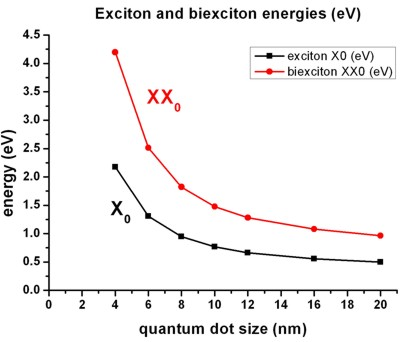• X0 is the energy when the exciton recombines.
The biexciton recombination energy (XX0 `-` X0) is the energy when the biexciton is subject to the transition from the biexciton ground state to the exciton ground state.
This energy is similar to the energy X0.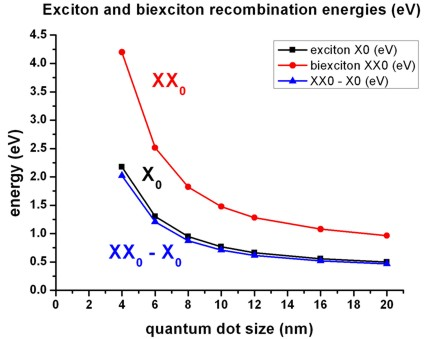• The binding energy of the biexciton is shown in comparison to the exciton binding energy.
Ee,0 and Eh,0 are the electron and hole one-particle energies (without excitonic corrections).• More details on the biexciton:``` \$numeric-control```

• Please help us to improve our tutorial. Send comments to``` support [at] nextnano.com```.